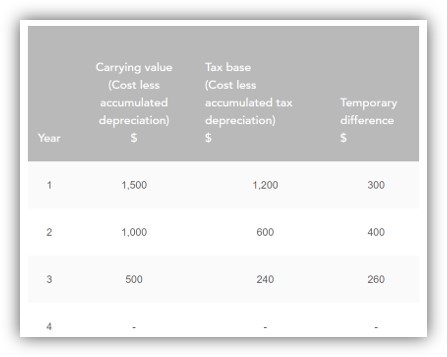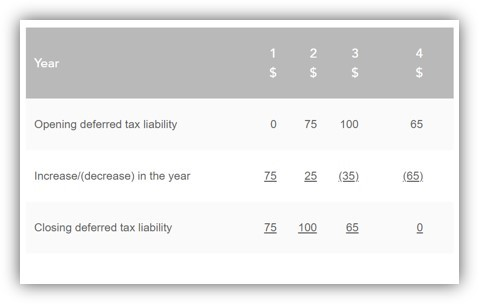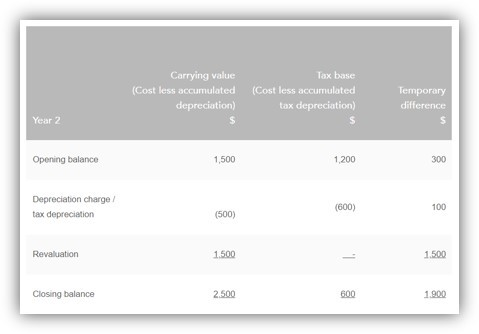# Deferred Tax

Deferred tax is a topic that is regularly tested in Financial Reporting (FR) and is often tested in further detail in Strategic Business Reporting (SBR). This article will consider the aspects of deferred tax that are relevant to FR.

## The Basics

Deferred tax is accounted for in accordance with IAS® 12, Income Taxes. It is important to note that references to ‘income tax’ here are to tax on company profits or losses rather than tax on an individual’s income. Tax related to companies may be referred to as ‘corporation tax’ or ‘corporate income tax’ in some jurisdictions.

In FR, deferred tax normally results in a liability being recognised within the statement of financial position. IAS 12 defines a deferred tax liability as being the amount of income tax payable in future periods in respect of taxable temporary differences. So, in simple terms, deferred tax is tax that is payable in the future. However, to understand this definition more fully, it is necessary to explain the term ‘temporary differences’.

Temporary differences are defined as being differences between the carrying amount of an asset or liability in the statement of financial position and its tax base (ie the amount attributed to that asset or liability for tax purposes).

Temporary differences may be either ‘taxable temporary differences’ or ‘deductible temporary differences’.

Taxable temporary differences are those on which tax will be charged in the future when the asset (or liability) is recovered (or settled).

Deductible temporary differences are those which will result in tax deductions or savings in the future when the asset (or liability) is recovered (or settled).

IAS 12 requires that a deferred tax liability is recorded in respect of all taxable temporary differences that exist at the year-end.

All of this terminology can be rather overwhelming and difficult to understand, so consider it alongside an example. Depreciable non-current assets are a typical deferred tax example used in FR to examine knowledge and understanding.

Within financial statements, non-current assets with a limited useful life are subject to depreciation. However, within the corresponding tax computations, non-current assets are subject to tax depreciation (sometimes known as ‘capital allowances’) at rates set within the relevant tax legislation. Where at the year-end the accumulated depreciation and the cumulative tax depreciation claimed are different, the carrying amount of the asset (cost less accumulated depreciation) will then be different to its tax base (cost less accumulated tax depreciation) and hence a temporary difference arises.

## EXAMPLE

A non-current asset with a cost of GBP2,000 was acquired at the start of year 1. It is being depreciated on a straight-line basis over four years, resulting in annual depreciation charges of GBP500. Therefore, a total of GBP2,000 of depreciation will be charged over the life of the asset. The tax depreciation granted by the tax authorities on this asset are:The table below shows the carrying amount of the asset, the tax base of the asset and therefore the temporary difference at the end of each year:As stated above, deferred tax liabilities arise on taxable temporary differences (i.e. those temporary differences that result in tax being payable in the future as the temporary difference reverses). So, how does the above example result in tax being payable in the future?

Entities pay income tax on their taxable profits. When determining taxable profits, the tax authorities start by taking the profit before tax (accounting profits) of an entity from their financial statements and then make various adjustments. For example, depreciation is considered a disallowable expense for taxation purposes but instead tax relief on asset expenditure (capital expenditure) is granted in the form of tax depreciation.

Therefore, taxable profits are arrived at by adding back depreciation and deducting tax depreciation from the accounting profits. Entities are then charged tax at the appropriate tax rate on these taxable profits.

In the above example, when the tax depreciation is greater than the depreciation expense in years 1 and 2, the entity has received tax relief early. This is good for cash flow in that it delays (i.e. defers) the payment of tax. However, the difference is only a temporary difference and so the tax will have to be paid in the future. In years 3 and 4, when the tax depreciation for the year is less than the depreciation charged, the entity is being charged additional tax and the temporary difference is reversing. Hence the temporary differences can be said to be taxable temporary differences.

Notice that overall, the accumulated depreciation and accumulated tax depreciation both equal GBP2,000 – the cost of the asset – so over the four-year period, there is no difference between the taxable profits and the profits per the financial statements. Where local tax legislation requires that tax depreciation is calculated on a reducing (diminishing) balance basis, then the asset may be fully depreciated before the full amount of tax depreciation has been claimed. This does not make a difference to the accounting required for deferred tax.

In this example, at the end of year 1 the entity has a temporary difference of GBP300, which will result in tax being payable in the future (in years 3 and 4). In accordance with the accruals concept, a liability is therefore recorded equal to the expected tax payable.

Assuming that the tax rate applicable to the company is 25%, the deferred tax liability that will be recognised at the end of year 1 is 25% x GBP300 = GBP75. This will be recorded by crediting (increasing) a deferred tax liability in the statement of financial position and debiting (increasing) the income tax expense in the statement of profit or loss.

By the end of year 2, the entity has a taxable temporary difference of GBP400 (i.e. the GBP300 bought forward from year 1, plus the additional difference of GBP100 arising in year 2). A liability is therefore now recorded equal to 25% x GBP400 = GBP100. Since there was a liability of GBP75 recorded at the end of year 1, the double entry that is recorded in year 2 is to credit (increase) the liability and debit (increase) the income tax expense by GBP25.

At the end of year 3, the entity’s taxable temporary differences have decreased to GBP260 since the company has now been charged tax on the difference of GBP140 (GBP500 depreciation - GBP360 tax depreciation). In other words, they are now adding back more depreciation in their tax computation than they are able to deduct in tax depreciation. Therefore, in the future, the tax payable will be 25% x GBP260 = GBP65. The deferred tax liability now needs to be reduced from GBP100 to GBP65 and so is debited (a decrease) by GBP35. Consequently, there is now a credit (a decrease) to the income tax expense of GBP35.

At the end of year 4, there are no taxable temporary differences since now the carrying amount of the asset is equal to its tax base. Therefore, the opening liability of GBP65 needs to be removed by a debit entry (a decrease) and hence there is a credit entry (a decrease) of GBP65 to the income tax expense. This can all be summarised in the following working:The movements in the liability are recorded in the statement of profit or loss as part of the income tax charge.

The closing figures are reported in the statement of financial position as part of the deferred tax liability.

The statement of profit or loss
As IAS 12 considers deferred tax from the perspective of temporary differences between the carrying amount and tax base of assets and liabilities, the standard can be said to focus on the statement of financial position. However, it is helpful to consider the effect on the statement of profit or loss.

Continuing with the previous example, suppose that the profit before tax of the entity for each of years 1 to 4 is GBP10,000 (after charging depreciation). Since the tax rate is 25%, it would then be logical to expect the income tax expense for each year to be GBP2,500. However, income tax is based on taxable profits, not on the accounting profits.

The taxable profits and current tax liability for each year could be calculated as in the table below:The current tax liability is recorded as part of the income tax expense. As we have seen in the example, accounting for deferred tax then results in a further increase or decrease in the income tax expense. Therefore, the final income tax expense for each year reported in the statement of profit or loss would be as follows:It can therefore be said that accounting for deferred tax is ensuring that the matching principle is applied. The income tax expense reported in each period is the tax consequences (i.e. tax charges less tax relief) of the items reported within profit in that period.

However, it should be noted that the tax consequences of transactions should be accounted for in the same way that the underlying transaction is accounted for. Therefore, if a transaction is accounted for outside of the statement of profit or loss (either in other comprehensive income or directly in equity), then the tax effect should also be recognised either in other comprehensive income or directly in equity as appropriate. See Example 2 for further details.

## Revaluations of non-current assets

Revaluations of non-current assets are a further example of a taxable temporary difference. When a non-current asset is revalued to its fair value within the financial statements, the revaluation gain is recorded in equity (revaluation surplus) and reported as other comprehensive income. While the carrying amount of the asset has increased, the tax base of the asset remains the same and so a temporary difference arises.

Tax will become payable on the gain when the asset is sold and so the temporary difference is taxable. Since the revaluation gain has been recognised within other comprehensive income and included as part of equity, the tax charge on the surplus is also recorded in other comprehensive income and reflected in the revaluation surplus balance in equity. Suppose that in Example 1, the asset is revalued to GBP2,500 at the end of year 2, as shown below:The carrying amount will now be GBP2,500 while the tax base remains at GBP600. This results in a temporary difference of GBP1,900, of which GBP1,500 relates to the revaluation gain. This gives rise to a deferred tax liability of GBP475 (25% x GBP1,900) at the year-end to report in the statement of financial position. The liability was GBP75 at the end of the prior year (Example 1) and thus there is an increase of GBP400 to record.

However, the increase in relation to the revaluation gain of GBP375 (25% x GBP1,500) will therefore reduce the total recorded in the revaluation surplus to GBP1,125 (GBP1,500 - GBP375). The revaluation gain itself can be presented in other comprehensive income net of tax or can be shown gross (i.e. without netting off the tax). If the gross revaluation gain is presented then the tax effect should be shown as a separate line item, aggregated with the tax effect of any other items of other comprehensive income presented gross.

The remaining increase in deferred tax of GBP25 will be charged to the statement of profit or loss as before. The overall journal entry required would be:

Dr Tax expense in Income Statement GBP25
Dr Revaluation reserve in equity GBP375
Cr Deferred tax liability in SFP GBP400